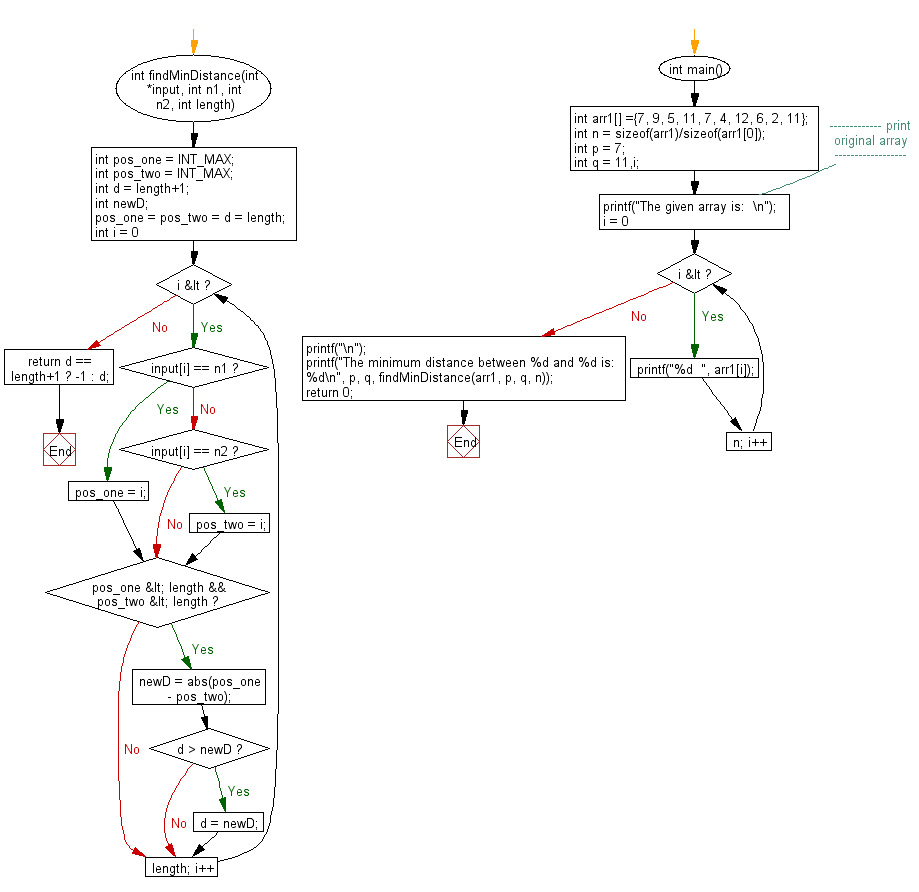﻿ C exercises: Find the minimum distance between two numbers in a given array - w3resource# C Exercises: Find the minimum distance between two numbers in a given array

## C Array: Exercise-84 with Solution

Write a program in C to find the minimum distance between two numbers in a given array.

Sample Solution:

C Code:

``````#include <stdio.h>
#include <stdlib.h>
#include <limits.h>

int findMinDistance(int *input, int n1, int n2, int length)
{
int pos_one = INT_MAX;
int pos_two = INT_MAX;
int d = length+1;
int newD;
pos_one = pos_two = d = length;

for (int i = 0; i < length; i++)
{
if (input[i] == n1)
pos_one = i;
else if (input[i] == n2)
pos_two = i;

if (pos_one < length && pos_two < length)
{
newD = abs(pos_one - pos_two);
if (d > newD)
d = newD;
}
}

return d == length+1 ? -1 : d;
}

int main()
{
int arr1[] ={7, 9, 5, 11, 7, 4, 12, 6, 2, 11};
int n = sizeof(arr1)/sizeof(arr1);
int p = 7;
int q = 11,i;
//------------- print original array ------------------
printf("The given array is:  \n");
for(i = 0; i < n; i++)
{
printf("%d  ", arr1[i]);
}
printf("\n");
//------------------------------------------------------
printf("The minimum distance between %d and %d is:  %d\n", p, q, findMinDistance(arr1, p, q, n));
return 0;
}
```
```

Sample Output:

```The given array is:
7  9  5  11  7  4  12  6  2  11
The minimum distance between 7 and 11 is:  1
```

Pictorial Presentation:Flowchart:C Programming Code Editor:

Improve this sample solution and post your code through Disqus.

﻿